# 3 Bags Full

## Slot Machine published 25 years ago by Aristocrat Leisure Industries Pty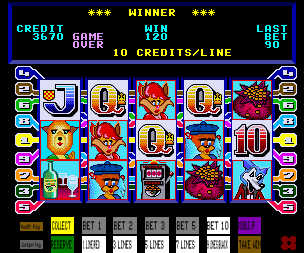## Listed and emulated in MAME !
Info
ROMs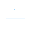(0)
Edit
Upload
### 3 Bags Full © 1995 Aristocrat.

A 5-reel video slot machine.

### TECHNICAL INFORMATION

1
Released on Aristocrat MKIV and MKV hardware with different CPU'S.

MKIV and Jubilee MKIV versions CPU: Motorola M6809
MKV version CPU: ARM250

Sound: 2x AY-3-8910A (@ 1.5MHz).

Screen Orientation: Horizontal
Screen Resolution: 320 x 224 pixels.
Refresh Rate: 60Hz.

The Jubilee MKIV version has a slightly different display with larger numbers, a coloured background replacing the rectangular border, and the reel graphics have been remade to look like the artwork.

### TRIVIA

Unlike most games, this game pays for two of a kind wins, with the exception of Money Bags and scattered Slot Machines.

### SCORING

Sheep substitutes for all symbols except scattered Slot Machine.

Paytable (Australian versions):
Sheep: 2 = 5, 3 = 100, 4 = 1000, 5 = 5000
Fox: 2 = 5, 3 = 20, 4 = 100, 5 = 1000
Cat: 2 = 5, 3 = 20, 4 = 100, 5 = 1000
Wolf: 2 = 5, 3 = 20, 4 = 100, 5 = 500
Glass: 2 = 5, 3 = 20, 4 = 100, 5 = 500
King: 2 = 2, 3 = 10, 4 = 25, 5 = 200
Queen: 2 = 2, 3 = 10, 4 = 25, 5 = 200
Jack: 2 = 2, 3 = 5, 4 = 20, 5 = 100
Ten: 2 = 2, 3 = 5, 4 = 20, 5 = 100
Money Bag: 3 = 5, 4 = 20, 5 = Feature
Slot Machine: 3 = 2, 4 = 10, 5 = 100

Paytable (New Zealand version):
Sheep: 2 = 5, 3 = 100, 4 = 600, 5 = 1000
Fox: 2 = 5, 3 = 20, 4 = 100, 5 = 800
Cat: 2 = 5, 3 = 20, 4 = 100, 5 = 800
Wolf: 2 = 5, 3 = 20, 4 = 100, 5 = 500
Glass: 2 = 5, 3 = 20, 4 = 100, 5 = 500
King: 2 = 2, 3 = 10, 4 = 25, 5 = 200
Queen: 2 = 2, 3 = 10, 4 = 25, 5 = 200
Jack: 2 = 2, 3 = 5, 4 = 20, 5 = 100
Ten: 2 = 2, 3 = 5, 4 = 20, 5 = 100
Money Bag: 3 = 5, 4 = 20, 5 = Feature
Slot Machine: 3 = 2, 4 = 15, 5 = 100

### TIPS AND TRICKS

When 5 Money Bags occur on a lit line, a second screen bonus appears. On the second screen, you can pick three items, which have varying amounts of credits; these credits are all added and is then multiplied by the current bet per line.

The Sheep also substitutes for Money Bags, so it doesn't have to be all 5 of the same item to trigger the feature.